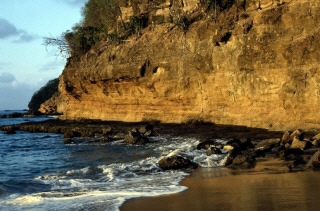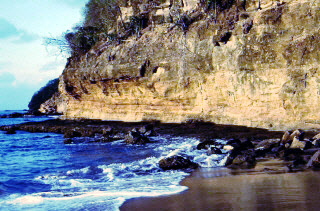﻿ Class | (Leadtools.ImageProcessing.Effects) | Raster, Medical, Document Help
←Select platform
In This Topic ▼

# MatchHistogramCommand Class

Summary
Transforms an image so that its histogram matches another images's histogram.

Syntax
C#
C++/CLI
Python
``public class MatchHistogramCommand : RasterCommand ``
````public: `
`   ref class MatchHistogramCommand : RasterCommand ````
``class MatchHistogramCommand(RasterCommand): ``

Remarks This command supports 8-bit grayscale and 24-bit color images only.

If the image to be transformed is 8-bit grayscale then the reference image must be 8-bit grayscale also.

If the image to be transformed is 24-bit color then the reference image can be 8-bit grayscale or 24-bit color.

Use the Run method to apply the matching on the image.

Match Histogram Function - BeforeMatch Histogram Function - AfterView additional platform support for this Match Histogram function.

Example
C#
````using Leadtools; `
`using Leadtools.Codecs; `
`using Leadtools.ImageProcessing.Effects; `
` `
` `
`public void MatchHistogramCommandExample() `
`{ `
`   //Load the two images `
`   RasterCodecs codecs = new RasterCodecs(); `
`   codecs.ThrowExceptionsOnInvalidImages = true; `
`   RasterImage RefBitmap = codecs.Load(Path.Combine(LEAD_VARS.ImagesDir, "cannon.jpg")); `
`   RasterImage Bitmap = codecs.Load(Path.Combine(LEAD_VARS.ImagesDir, "cannon_HistogramMatchingSample.jpg")); `
` `
`   //Prepare the command `
`   MatchHistogramCommand command = new MatchHistogramCommand(RefBitmap); `
` `
`   //Apply `
`   command.Run(Bitmap); `
`} `
` `
`static class LEAD_VARS `
`{ `
`   public const string ImagesDir = @"C:\LEADTOOLS22\Resources\Images"; `
`} ````
Requirements
Help Version 22.0.2023.3.31